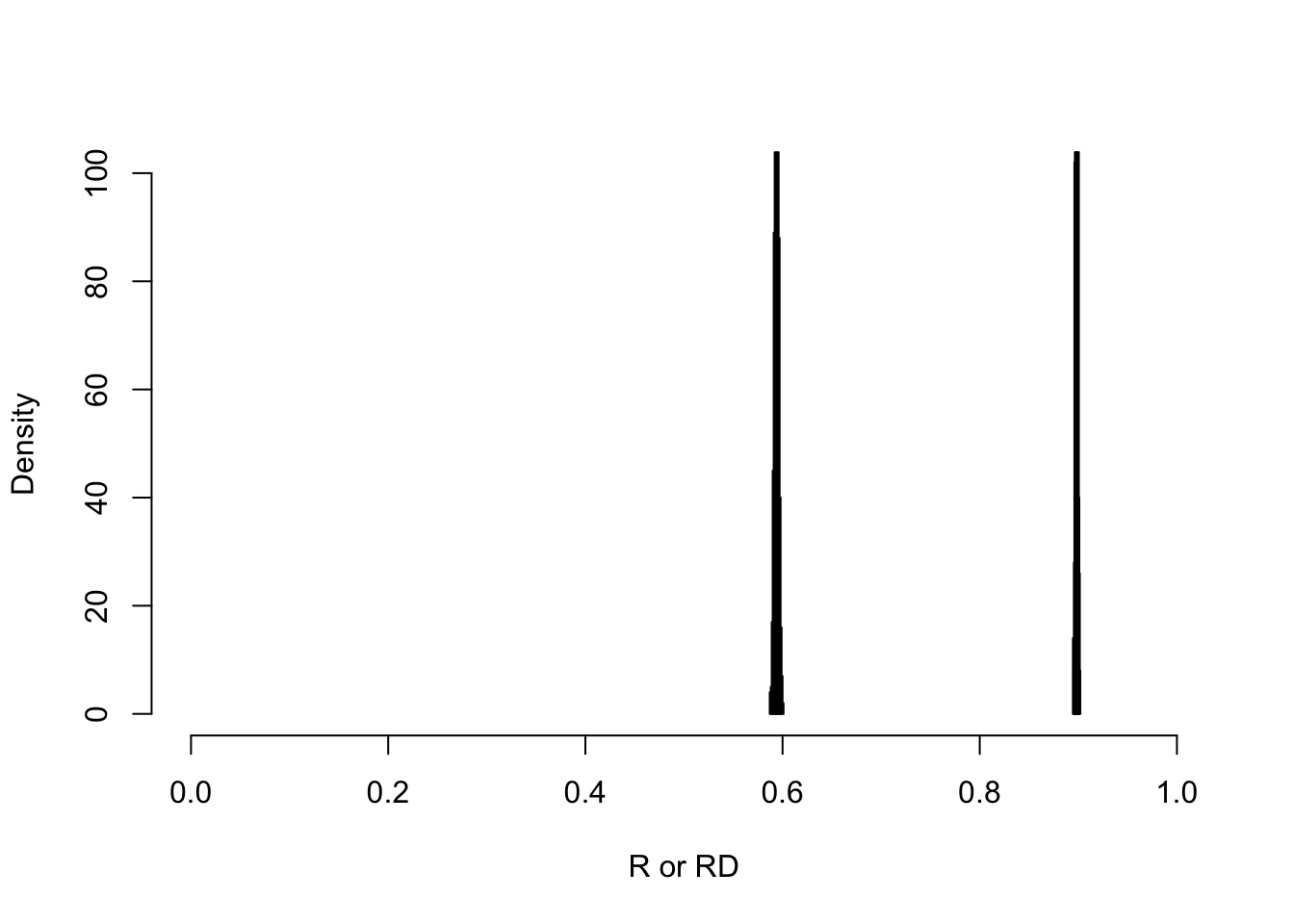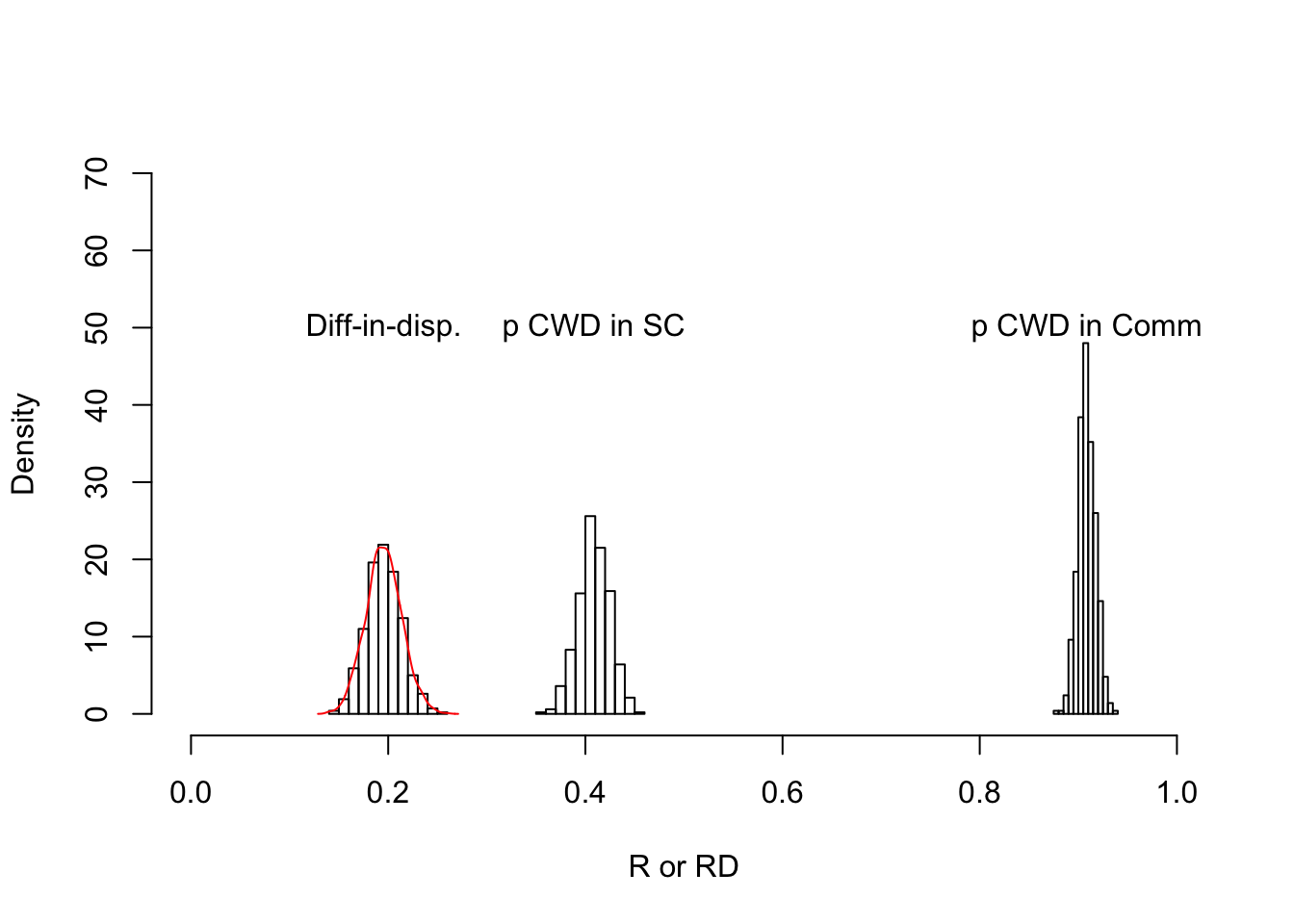## Set the design parameters for the study

The first stage is to set the number of disabled and non-disabled children that will be recruited overall, and the number of clusters.

library(openxlsx)
library(dplyr)
# Load the number of people enumerated in each cluster
N <- openxlsx::read.xlsx('Cluster Population CE1.xlsx')
N[,3] <- round(N[,2]*(2000/sum(N[,2])),0) # back-calc for the proportion of CWD in each cluster
N[,4] <- round(N[,2]*(2000/(sum(N[,2]*0.05))),0) # back-calc for the total children in each cluster
colnames(N) <- c('cluster','N','N_cwd','N_c')
head(N)
##   cluster    N N_cwd  N_c
## 1       1 2567    42  843
## 2       2 3471    57 1140
## 3       3 2165    36  711
## 4       4 2405    39  790
## 5       5 2395    39  786
## 6       6 3032    50  996
# n <- N[,3] # total number of disabled children
#
# d <- matrix(,nrow=n*2, ncol=4) # Create data
# d[,1] <- rep(c(0,1),times=c(n,n)) # Code disabled, and non-disabled (1,0)
# c <- 40 # total number of clusters
# d[,2] <- rep(rep(seq(1,c,1), times=rep(n/c, times=c)),times=2)

## Set assummed population-level parameters

Next, we set the population-level parameters, e.g. the ‘true’ parameter values, for each cluster. In this case, that means the true proportion attending school for disabled and non-disabled children.

# Enrolment
e0 <- c(.7) # enrolment of all children
e1 <- c(.5) # enrolment of disabled children
k <- c(.01) # Set intercluster coefficient of variation in enrollment levels
var_b0 <- k/e0 # Calculate the variance of the cluster proportions enrolled
var_b1 <- k/e1 # Calculate the variance of the cluster proportions enrolled
# Fix true cluster-level means of school enrollment
rnorm_0_1 <- function(p, v){
r <- 1
while (r>=1 | r<=0){
r <- round(rnorm(1, p, v^.5),2)
}
return(r)
}

N$e_0 <- apply(N, 1, function(x) rnorm_0_1(e0, var_b0)) N$e_1 <- apply(N, 1, function(x) rnorm_0_1(e1, var_b1))

# True relationship between enrollment and treatment in school-treatment arm
a0 <- c(.9) # 90% of enrolled children are in school and treated
a1 <- c(.8) # 80% of enrolled children with disabilities are in school and treated
# True proportions treated by the intervention
k <- c(.01) # Set intercluster coefficient of variation in attendance if enrolled
var_b0 <- k/a0 # Calculate the variance of the cluster attendance if enrolled
var_b1 <- k/a1 # Calculate the variance of the cluster attendance if enrolled
# Fix the true cluster-level means of the attendance at school if enrolled
N$a_0 <- apply(N, 1, function(x) rnorm_0_1(a0, var_b0)) N$a_1 <- apply(N, 1, function(x) rnorm_0_1(a1, var_b1))

# Allocate half clusters to treatment and half to control
N$allocation <- sample(c(rep(0,length.out=(nrow(N)/2)),rep(1,length.out=nrow(N)/2))) # Proportion treated in each cluster == # School-only arm: proportion enrolled * proportion in school if enrolled N$t_0 <- N$e_0 * N$a_0 # all children
N$t_1 <- N$e_1 * N$a_1 # children with disabilities # Community arm: set at 98% with small se t_comm <- .98 var_t_comm <- k/t_comm N$t_1[N$allocation == 1] <- apply(N[N$allocation == 1,], 1, function(x) rnorm_0_1(t_comm, var_t_comm))
N$t_0[N$allocation == 1] <- apply(N[N$allocation == 1,], 1, function(x) rnorm_0_1(t_comm, var_t_comm)) ## Generate enrolment outcome Finally, we take 1000 samples from the population, essentially simulating doing the planned study 1000 times. # Number of treated children overall, and number of treated disabled children i <- 1 t_0 <- matrix(, ncol=0, nrow=nrow(N)) t_1 <- matrix(, ncol=0, nrow=nrow(N)) while (i<=1000){ t_0 <- cbind(t_0, apply(N, 1, function(x) sum(sample(c(0,1),size=x,prob=c(1-x['t_0'],x['t_0']),replace=T)))) t_1 <- cbind(t_1, apply(N, 1, function(x) sum(sample(c(0,1),size=x,prob=c(1-x['t_1'],x['t_1']),replace=T)))) i <- i+1 } ## Conduct analysis on simulated data Using the 1000 simulations, we can conduct whatever analysis we want and see how the estiamtes vary from one simulated sample to the next. For example, we can estimate the proportion attending and the difference between the proportions for disabled and non disabled children. # Produce proportions treated in CWD and in children without disabilities # First need to subtract the number od disabled children treated from the number of non-disabled children treated t_0 <- t_0 - t_1 # And the denominators N_0 <- N[,2] - N[,3] # Calculate the proportions t_0_p <- t_0 / N_0 t_1_p <- t_1 / N[,3] # Append the allocation t_0_p <- cbind(t_0_p, N$allocation)
t_1_p <- cbind(t_1_p, N$allocation) # Disparity between all children and CWD diff_p <- t_0_p[,1:(ncol(t_0_p)-1)] - t_1_p[,1:(ncol(t_1_p)-1)] diff_p <- cbind(diff_p, N$allocation)

# Calculate sample enrolment figures
p0_sc   <- as.data.frame(t_0_p) %>% filter(V1001==0) %>% select(-V1001) %>% apply(2, mean)
p1_sc   <- as.data.frame(t_1_p) %>% filter(V1001==0) %>% select(-V1001) %>% apply(2, mean)
p0_comm <- as.data.frame(t_0_p) %>% filter(V1001==1) %>% select(-V1001) %>% apply(2, mean)
p1_comm <- as.data.frame(t_1_p) %>% filter(V1001==1) %>% select(-V1001) %>% apply(2, mean)

# Median disparity in each arm
diff_sc <- as.data.frame(diff_p) %>% filter(V1001==0) %>% select(-V1001) %>% apply(2, mean)
diff_comm <- as.data.frame(diff_p) %>% filter(V1001==1) %>% select(-V1001) %>% apply(2, mean)

# Difference in the disparity between the arms
diff_in_disp <- diff_sc - diff_comm

We can visualise the level of precision by plotting the results from all 1000 samples. Here we have the proportion in school for children with disabilities, children without disabilities (controls), and the difference between these for each run of the simulation.

## Proportions treated in children without disability

hist(p0_sc, xlim=c(0,1),ylim=c(0,100), main='', xlab='R or RD', freq=F)
hist(p0_comm, add =T, freq=F)## Proportions treated in children with disability

hist(p1_sc, xlim=c(0,1),ylim=c(0,70), main='',
xlab='R or RD', freq=F)
hist(p1_comm, add =T, freq=F)
hist(diff_in_disp, add=T, freq=F)
lines(density(diff_in_disp),col='red')
text(x=c(mean(diff_in_disp),mean(p1_sc),mean(p1_comm)),
y=c(50),labels=c('Diff-in-disp.', 'p CWD in SC','p CWD in Comm'))